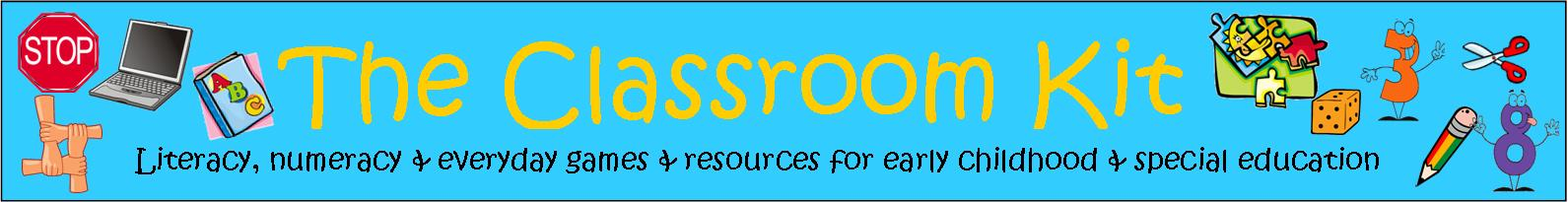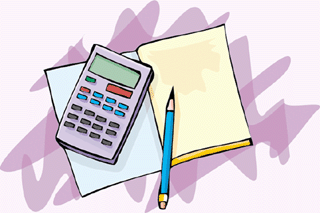# Numeracy WorksheetsNumeracy worksheets or blackline masters, are a useful way to teach specific mathematical concepts to a group. These ones include some repetitive activities, such as simple addition and subtraction sums, some templates for use in a variety of activities, such as venn diagrams and bar graphs, some tasks requiring mathematical understandings, such as exploring mass and length, and some game-like activities.

While some worksheets have been created in colour, it is not necessary for them to be reproduced in colour unless otherwise stated.

Some sets of worksheets have been included, that allow concepts to be built gradually over several weeks of working on a unit. These have been numbered to assist in distinguishing between each one.

Some sets of worksheets have been produced to incorporate thematic units of study, or alphabet studies (to be used as "letter of the week activities").

Stages of learning numeracy concepts
Number Worksheets
Measurement Worksheets
Pattern Worksheets
Spatial Awareness Worksheets
Chance and Probability
Data Collection and Analysis (Working with Graphs)